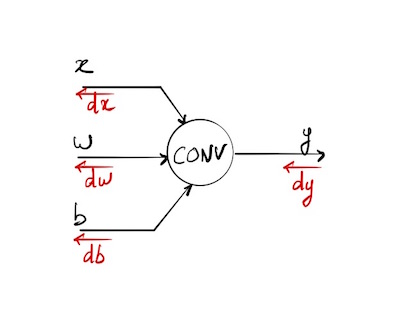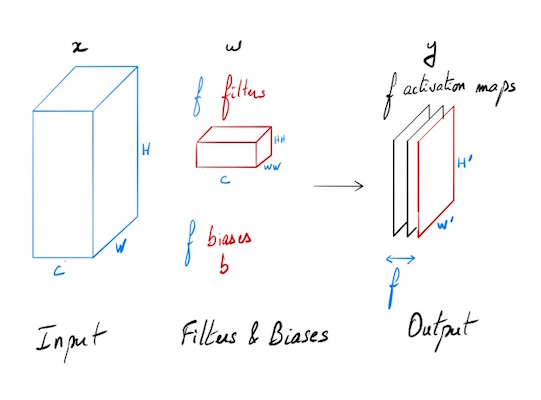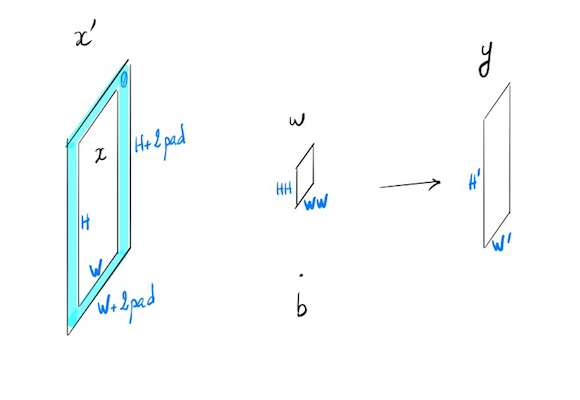Backpropagation in a convolutional layer

Working on the Stanford course CS231n: Convolutional Neural Networks for Visual Recognition. I did not manage to find a complete explanation of how backprop math is working. So here is a post detailing step by step how this key element of Convnet is dealing with backprop.## Introduction

### Motivation

The aim of this post is to detail how gradient backpropagation is working in a convolutional layer of a neural network. Typically the output of this layer will be the input of a chosen activation function (relufor instance). We are making the assumption that we are given the gradient dy backpropagated from this activation function. As I was unable to find on the web a complete, detailed, and “simple” explanation of how it works. I decided to do the maths, trying to understand step by step how it’s working on simple examples before generalizing. Before further reading, you should be familiar with neural networks, and especially forward pass, backpropagation of gradient in a computational graph and basic linear algebra with tensors.### Notations

* will refer to the convolution of 2 tensors in the case of a neural network (an input x and a filter w).

• When xand w are matrices:
• if xand w share the same shape, x*w will be a scalar equal to the sum across the results of the element-wise multiplication between the arrays.
• if wis smaller the x, we will obtain an activation map y where each value is the predefined convolution operation of a sub-region of x with the sizes of w. This sub-region activated by the filter is sliding all across the input array x.
• if xand w have more than 2 dimensions, we are considering the last 3 ones for the convolution, and the last 2 ones for the highlighted sliding area (we just add one depth to our matrix)

Notations and variables are the same as the ones used in the excellent Stanford course on convolutional neural networks for visual recognition and especially the ones of assignment 2. Details on convolutional layer and forward pass will be found in this video and an instance of a naive implementation of the forward pass post.### Goal

Our goal is to find out how gradient is propagating backwards in a convolutional layer. The forward pass is defined like this:

The input consists of N data points, each with C channels, height H and width W. We convolve each input with F different filters, where each filter spans all C channels and has height HH and width WW.

Input:

• x: Input data of shape (N, C, H, W)
• w: Filter weights of shape (F, C, HH, WW)
• b: Biases, of shape (F,)
• conv_param: A dictionary with the following keys:
• ‘stride’: The number of pixels between adjacent receptive fields in the horizontal and vertical directions.
• ‘pad’: The number of pixels that will be used to zero-pad the input.

During padding, ‘pad’ zeros should be placed symmetrically (i.e equally on both sides) along the height and width axes of the input.

Returns a tuple of:

• out: Output data, of shape (N, F, H’, W’) where H’ and W’ are given by H’ = 1 + (H + 2 * pad - HH) / stride W’ = 1 + (W + 2 * pad - WW) / stride
• cache: (x, w, b, conv_param)

### Forward pass

#### Generic case (simplified with N=1, C=1, F=1)

N=1 one input, C=1 one channel, F=1 one filter.• $x$ : $H \times W$
• $x’ = x$ with padding
• $w$ : $HH \times WW$
• $b$ bias : scalar
• $y$ : $H’\times W’$
• stride $s$

$\forall (i,j) \in [1,H’] \times [1,W’]$

### Backpropagation

We know:

$dy = \left(\frac{\partial L}{\partial y_{ij}}\right)$

We want to compute $dx$, $dw$ and $db$, partial derivatives of our cost funcion L. We suppose that the gradient of this function has been backpropagated till y.

## Trivial case: input x is a vector (1 dimension)

We are looking for an intuition of how it works on an easy setup and later on we will try to generalize.

### Backpropagation

We know the gradient of our cost function L with respect to y:

This can be written with the Jacobian notation:

dy and y share the same shape:

We are looking for

#### db

Using the chain rule and the forward pass formula (1), we can write:

#### dw

We can notice that dw is a convolution of the input x with a filter dy. Let’s see if it’s still valid with a added dimension.

#### dx

Once again, we have a convolution. A little bit more complex this time. We should consider an input dy with a 0-padding of size 1 convolved with an “inverted” filter w like $(w_2, w_1)$

Next step will be to have a look on how it works on small matrices.

## Input x is a matrix (2 dimensions)

### Output

Once again, we will choose the easiest case: stride = 1 and no padding. Shape of y will be (3,3)

We will have:

Written with subscripts:

### Backpropagation

We know:

#### db

Using the Einstein convention to alleviate the formulas (when an index variable appears twice in a multiplication, it implies summation of that term over all the values of the index)

Summation on i and j. And we have:

#### dw

We are looking for

Using the formula (4) we have:

All terms

Except for $(k,l) = (m,n)$ where it’s 1, case occurring just once in the double sum.

Hence:

Using formula (3) we now have:

If we compare this equation with formula (1) giving the result of a convolution, we can distinguish a similar pattern where dy is a filter applied on an input x.

#### dx

Using the chaine rule as we did for (5), we have:

This time, we are looking for

Using equation (4):

We now have:

In our example, range sets for indices are:

When we set $k=m-i+1$, we are going to be out of the defined boundaries:

In order to keep confidence in formula (7), we choose to extend the definition of matrix $w$ with $0$ values as soon as indices will go out of the defined range.

Once again in the double sum (7), we only have once partial derivative of x equals 1. So, using (7) and (8):

where $w$ is our 0-extended initial filter

Injecting this formula in (6) we obtain:

Lets visualize it on several chosen values for the indices.

Using $*$ notation for convolution, we have:

As $dy$ remain the same, we will only look at the values of indices of w. For $dx_{22}$, range for w: $3-i,3-j$

We now have a convolution between dy and a w’ matrix defined by:

Another instance in order to see what’s happening. $dx_{43}$, w : $4-i,3-j$

Last one $dx_{44}$

We do see poping up an “inverted filter” w’. This time we have a convolution between an input $dy$ with a 0-padding border of size 1 and a filter w’ slidding with a stride of 1.

## Taking depths into account

Things are becoming slightly more complex when we try to take depth into account (C channels for input x, and F distinct filters for w)

Inputs

• x: shape (C, H, W)
• w: filter’s weights shape (F, C, HH, WW)
• b: shape (F,)

Outputs:

• y: shape (F, H’, W’)

Maths formulas see many indices emerging, making them more difficult to read. The forward pass formula in our example will be:

### db

db computation remains easy as each $b_f$ is related to an activation map $y_f$:

### dw

Using (10), as the double sum does not use dy indices, we can write:

### Algorithm

Now that we have the intuition of how it’s working, we choose not to write the entire set of equations (which can be pretty tedious), but we’ll use what has been coded for the forward pass, and playing with dimensions try to code the backprop for each gradient. Fortunately we can compute a numerical value of the gradient to check our implementation. This implementation is only valid for a stride=1, thing are becoming slightly more complex with a distinct stride, and another approach is needed. Maybe for another post!

This first solution is only valid in a stride = 1 case

Testing conv_backward_naive function
dx error:  7.489787768926947e-09
dw error:  1.381022780971562e-10
db error:  1.1299800330640326e-10


Almost 0 each time, everything seems tobe OK! :)

#### Généralisation pour tout stride - Nouvel article?

Dans cette version on regarde la contribution de chaque volume d’entrée au résultat y et on rétropropage en calculant des produits de convolution entre volumes de tailles identiques. Dans ce cas on a dx_input_volume = dy[i,j] . w (. est ici un simple produit). On cumule ensuite toutes les contributions dx qui participent aux mêmes indices. Finalement c’est beaucoup plus simple dans la mise en oeuvre que la version mathématique appliquée sur l’ensemble des valeurs de x.

Voici une version sans vectorisation, particulièrement lente, mais cela permet de voir comment les choses se mettent en place. Que ce soit pour dw ou dx, on se limite pour le coeur du calcul à l’influence du produit de convolution de deux éléments de taille identique (qui donnent un scalaire), et on cumule ensuite lors des déplacements du filtre sur l’entrée x pendant la propagation.

pour x et w de mêmes dimensions, y est un scalaire:

Comments are welcome to improve this post, feel free to contact me!